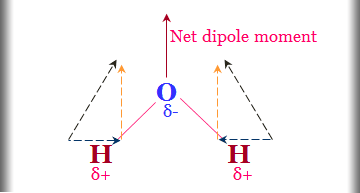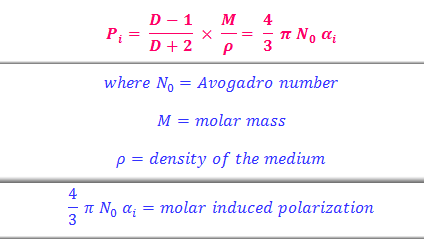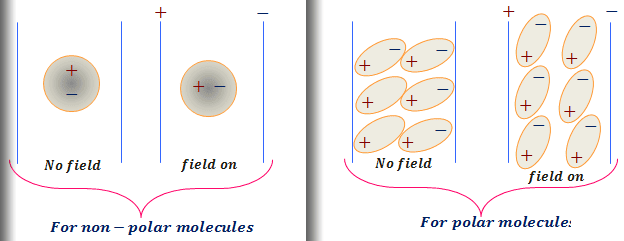# Bond polarity and dipole moments

### Study polarity of the bond and dipole moments

Evaluation and interpretation of the dipole moment of covalent molecules provide an important tool in the attack of molecular structure. It helps to determine the size and shape of the molecules, spatial arrangements of bonds, bond polarity, residue charge on the atoms of the molecules.

The molecules are composed of partially charged nuclei and negatively charged electrons distributed in space. The structural arrangement of these bonds is different in different molecules.

When the center of gravity of the positive charge due to coincides with the center of gravity of the negative charge due to electrons, the molecules become non-polar.
Hydrogen, carbon dioxide, boron trichloride, phosphorus pentachloride, benzine are the example of non-polar molecules.

When the center of gravity of the positive charge does not coincide with the center of gravity of the negative charge, polarity arises in the molecules and the molecules are called polar.
Hydrogen chloride, water, ammonia, methyl chloride, chlorobenzene are examples of polar molecules.

#### What is the dipole moment of a molecule?

The polarity of a bond in a molecule has quantified a term, called dipole moment (µ).

If +q amount of charge separates at the positive charge center, -q will be accumulated at the negative charge center of the molecule and l is the distance between two centers of the molecule.

∴ Dipole moment, µ = q × l
Non-polar molecules, l=0 and hence µ=0.

Higher the value of µ of a molecule, higher will be its polarity.

#### The polarity of bond in hydrochloric acid

In hydrochloric acid, due to the greater electronegativity of a chlorine atom, the bonding electron pair shifted towards chlorine atom. Thus chlorine atom acquires a small negative charge and hydrogen atom acquires a small positive charge. l is the distance of the charge separation usually taken in bond length.

∴ µ = q × l

The dipole moment is a vector quantity and it has both, magnitude and direction. The direction is represented by an arrow pointing towards the negative end. The length of the arrow is directly proportional to the magnitude of µ.Polarity of bond
Problem
P - F, S - F, Cl - F, and F - F which of the following compound has the lowest dipole moment?

The electronegativity difference between the two F atoms is zero. Thus the dipole moment of the fluorine molecule is zero.

#### CGS and SI unit of the dipole moment

In the CGS system, the charge expressed as esu and the length in cm. Thus the unit of dipole moment is esu cm.
The charge in order of 10⁻¹⁰ esu and distance of separation of charge in order of 10⁻⁸ cm.
∴ Order of dipole moment = 10⁻¹⁰ × 10⁻⁸
= 10⁻¹⁸ esu cm.
This magnitude is known as Debye or simply D.

∴ 1 Debye = 10⁻¹⁸ esu cm.

The dipole moment of hydrochloric acid = 1.03 D = 1.03 × 10⁻¹⁸ esu cm.

In the SI system, the charge is expressed in coulomb and length is a meter.

SI unit of dipole moment = coulomb × meter (c × m).

Problem
How can convert 1 Debye to the coulomb meter?

Solution
Dipole moment = q × l.

∴ The dipole moment of a molecule in the CGS unit
= 4.8 × 10⁻¹⁰ × 10⁻⁸ esu cm
= 4.8 D.

The dipole moment of a molecule in the SI unit
1.6 × 10⁻¹⁹ × 10⁻¹⁰ coulombs × meter
= 1.6 × 10⁻³⁰ C × m.

∴ 4.8 Debye = 1.6 ×10⁻³⁰ coulomb meter
or, 1 Debye = (1.6 × 10⁻³⁰)/4.8 coulomb meter
= 3.336 × 10⁻³⁰ coulomb meter.

#### The dimension of the dipole moment

Unit of dipole moment = unit of charge × unit of length.
∴ CGS unit of µ = esu × cm.

Coulomb’s Law, F = q₁q₂/Dr²
∴ (esu)² = dyne × cm² = gm cm sec⁻² × cm²
or, esu = gm1/2 sec⁻¹ cm3/2

∴ Dimension of µ = M1/2 T-1 L5/2

### What is the polarization?

When a non-polar substance placed between two parallel plates and an electric field applied, the field tends to attract the negatively charged electrons towards the positive plate and positive charge towards a negative plate.

Under this condition, there will be the electrical distortion of the molecule and an electric dipole is created. Such distortion in a molecule called the electric polarization.

Polarization, however, disappears as soon as the field is withdrawn and the molecule comes back to its original state.

#### Induced polarization of a molecule

Induced polarization (Pi) and the electric dipole created in the molecule due to the presence of the electric field called induced dipole moment (μi).

The induced dipole moment or simply the induced moment is directly proportional to the strength of the electric field applied(F).
∴ μi ∝ F
When F is low, otherwise, hyperpolarization may occur.
μi = αi F
where αi is proportionality constant called induced polarizability of the molecule.

Induced dipole moment measures the case with which the electronic configuration of the molecule can be distorted by an applied electric field. It may also be defined as the amount of induced moment in the molecule when the unit field strength is applied. The polarizability has the dimension of the volume.

αi = μi/F
∴ Dimension of αi = (esu × cm)/(esu × cm⁻²)
= cm³.

It can also be shown that, αi = r³ where r = radius of the molecule assuming it to have a spherical shape.

For atoms also, distortion occurs when it is placed between the two charged plates. The polarizability of the atom increases with the atomic size, atomic number and the case of ionization energy. Thus atom behaves like a dipole and this dipole moment is induced by the applied electric field.

#### Clausius Mossotti equation from electromagnetic theory

Clausius Mossotti derived from electromagnetic theory, a relation between the polarizability and the dielectric constant of the non-polar medium between two plates.Clausius Mossotti equation
Study online for schools and college-level
It gives the distortion produced in the 1 mole of the substance by a unit electric field. Induced polarizability is constant for the molecule and independent of temperature. Hence induced dipole moment is also constant for the molecule and independent of temperature.

D = dielectric constant of the medium = C/C₀
where C = capacitance of the condenser containing the substance and C₀ is the vacuum.
D is the dimensionless quantity and it is unity for vacuum. For other substances, it is greater than unity.

The induced dipole moment of the substance can be calculated by measuring dielectric constant, density (ρ) and knowing the molar mass (M) of the substance.

### Polar bonding molecules - Debye modification

Polar bonding molecules like methyl chloride, water, hydrogen fluoride, etc, molar polarization not constant but decreases with temperature.

Clausius Mossotti equation fails very badly for polar bonding molecules. The reason for the failure was put forward by P Debye.

According to him, when an electric field is applied between two parallel plates containing polar molecules (gas molecules), two effects will occur.

#### Induced polarization of molecules

The field will tend to induced polarization in the molecules and the induced polarization in the molecules.
∴ Pi= (4/3) π N₀ αi

#### Orientation polarization of molecules

The dipolar molecules will be oriented in the field producing a net dipole moment in the direction of the field.
∴ P₀ = (4/3) π N₀ α₀
where α₀ = orientation polarisability.
Debye calculated the value of α₀ = μ²/3KT

Considering the two tendencies that polar molecules tend to orient in the direction of the applied field (applied) and thermal orientation tends to destroy the alignment of the molecules.Polarization of bond

#### Total polarization of bond

For the polar bonding molecules, the total polarization,
Pt = Pi + P₀Debye equation

#### Temperature dependence on polarizability

A polar bond is fixed and unable to orient in the fixed direction, the orientation polarization has taken zero. This is true for the condensed system where strong intermolecular forces prevent the free rotation of the molecules.

When the temperature is very high tending to infinity.

1/T = 0 and Pt = 0
∴ Pt = Pi

At high temperatures, the polar molecules rotate at such high speed that orientation polarization vanishes.

Study polarity of the bond and dipole moments, SI unit of dipole bond moment, polarization, temperature dependence on polarizability in chemistry